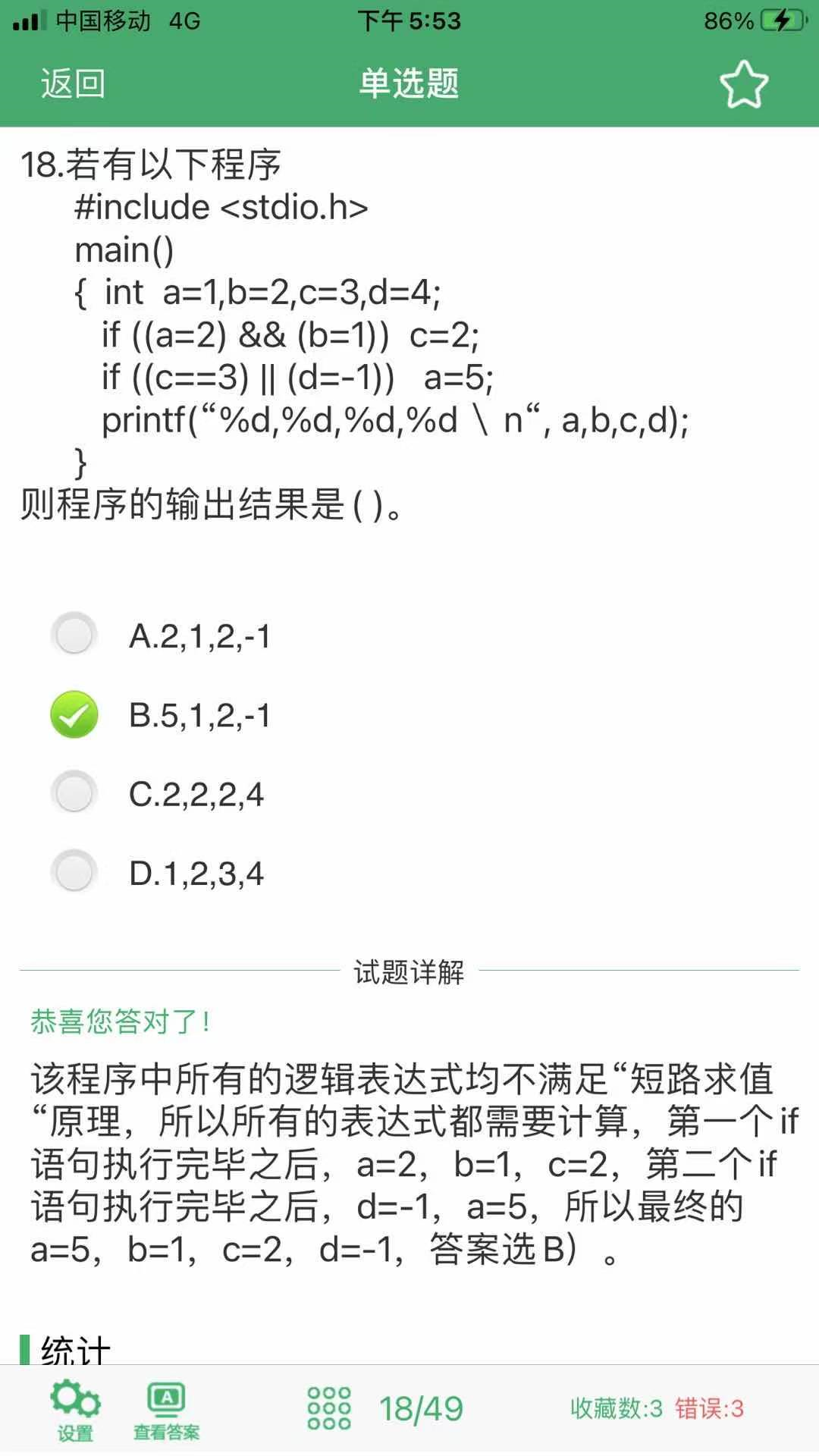2021-02-26 17:59

# 为什么在这种情况下不满足短路的原理？c==3不是已经true了吗，为什么后面的d=-1还要执行呢？

5• 点赞
• 写回答
• 关注问题
• 收藏
• 复制链接分享
• 邀请回答

#### 3条回答

• a = 2 和 b =1 都是赋值语句，所以值都是true, 就会执行c=2, 下面的 c == 3自然就是false了

点赞 1 评论 复制链接分享
• 我不是特别看的懂这个不过代码基本都差不多

你看第一步a=1,b=2,3=3,d=4 这一步是已经把四个参数的值给声明好了

第二步if((a=2)&&(b=1)){c=2}  问题主要出在这一步，a=2和b=1 不是判断，而是赋值，相当于重写了a和b的值，你学的这个应该是赋值为true吧

既然a=2为true并且b=1为true，那么就执行c=2，将c的值改为2.注意这时候c就已经不是3了（这时候你要记住a被赋值为2，b被赋值为1，c被赋值为2了）

然后是第三步if((c==3)||(d=-1)){a=5}  首先你要知道c==3已经是错误的了，因为这时候c的值经过第二步已经是2了，||是一个有一个为true就为true，d=-1又是一个赋值，然后跟你你们那个赋值为true的逻辑，表示这个判断是false||true，那么结果就是true，执行a=5的赋值

既然这样你想一下，在第三步的时候判断结果是true，执行a=5,判断里d=-1.。     第二步的时候a=2在第三步的时候被重写等于5了，所以不算他，b=1  然后执行了c=2的操作     最终结果就是a=5  b=1 c=2 d=-1

对不住兄弟，我没干过这种a=2为true的操作，所以只能说我根据结果和底下的问答，自己理解的，不对勿喷

点赞 评论 复制链接分享
• 第一步之后c=2了，c!=3的，所以会执行d=-1

点赞 1 评论 复制链接分享